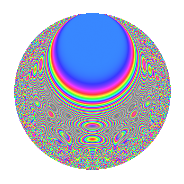Properties

 Label 6031.2.a.eLevel 6031 Weight 2 Character orbit 6031.a Self dual Yes Analytic conductor 48.158 Analytic rank 0 Dimension 134 CM No

Related objects

Newspace parameters

 Level: $$N$$ = $$6031 = 37 \cdot 163$$ Weight: $$k$$ = $$2$$ Character orbit: $$[\chi]$$ = 6031.a (trivial)

Newform invariants

 Self dual: Yes Analytic conductor: $$48.157777459$$ Analytic rank: $$0$$ Dimension: $$134$$ Fricke sign: $$-1$$ Sato-Tate group: $\mathrm{SU}(2)$

$q$-expansion

The dimension is sufficiently large that we do not compute an algebraic $$q$$-expansion, but we have computed the trace expansion.

 $$\operatorname{Tr}(f)(q) =$$ $$134q$$ $$\mathstrut +\mathstrut 9q^{2}$$ $$\mathstrut +\mathstrut 7q^{3}$$ $$\mathstrut +\mathstrut 149q^{4}$$ $$\mathstrut +\mathstrut 22q^{5}$$ $$\mathstrut +\mathstrut 20q^{6}$$ $$\mathstrut +\mathstrut 11q^{7}$$ $$\mathstrut +\mathstrut 27q^{8}$$ $$\mathstrut +\mathstrut 181q^{9}$$ $$\mathstrut +\mathstrut O(q^{10})$$ $$\operatorname{Tr}(f)(q) =$$ $$134q$$ $$\mathstrut +\mathstrut 9q^{2}$$ $$\mathstrut +\mathstrut 7q^{3}$$ $$\mathstrut +\mathstrut 149q^{4}$$ $$\mathstrut +\mathstrut 22q^{5}$$ $$\mathstrut +\mathstrut 20q^{6}$$ $$\mathstrut +\mathstrut 11q^{7}$$ $$\mathstrut +\mathstrut 27q^{8}$$ $$\mathstrut +\mathstrut 181q^{9}$$ $$\mathstrut +\mathstrut 15q^{10}$$ $$\mathstrut +\mathstrut 20q^{11}$$ $$\mathstrut +\mathstrut 28q^{12}$$ $$\mathstrut +\mathstrut 11q^{13}$$ $$\mathstrut +\mathstrut 17q^{14}$$ $$\mathstrut -\mathstrut 13q^{15}$$ $$\mathstrut +\mathstrut 143q^{16}$$ $$\mathstrut +\mathstrut 76q^{17}$$ $$\mathstrut +\mathstrut 23q^{18}$$ $$\mathstrut +\mathstrut 15q^{19}$$ $$\mathstrut +\mathstrut 67q^{20}$$ $$\mathstrut +\mathstrut 63q^{21}$$ $$\mathstrut +\mathstrut 2q^{22}$$ $$\mathstrut +\mathstrut 22q^{23}$$ $$\mathstrut +\mathstrut 33q^{24}$$ $$\mathstrut +\mathstrut 160q^{25}$$ $$\mathstrut +\mathstrut 65q^{26}$$ $$\mathstrut +\mathstrut 31q^{27}$$ $$\mathstrut +\mathstrut 10q^{28}$$ $$\mathstrut +\mathstrut 73q^{29}$$ $$\mathstrut +\mathstrut 20q^{30}$$ $$\mathstrut +\mathstrut 10q^{31}$$ $$\mathstrut +\mathstrut 53q^{32}$$ $$\mathstrut +\mathstrut 72q^{33}$$ $$\mathstrut -\mathstrut 7q^{34}$$ $$\mathstrut +\mathstrut 52q^{35}$$ $$\mathstrut +\mathstrut 201q^{36}$$ $$\mathstrut +\mathstrut 134q^{37}$$ $$\mathstrut +\mathstrut 70q^{38}$$ $$\mathstrut +\mathstrut 6q^{39}$$ $$\mathstrut +\mathstrut 11q^{40}$$ $$\mathstrut +\mathstrut 182q^{41}$$ $$\mathstrut -\mathstrut 15q^{42}$$ $$\mathstrut +\mathstrut 12q^{43}$$ $$\mathstrut +\mathstrut 33q^{44}$$ $$\mathstrut +\mathstrut 29q^{45}$$ $$\mathstrut +\mathstrut 24q^{46}$$ $$\mathstrut +\mathstrut 80q^{47}$$ $$\mathstrut +\mathstrut 21q^{48}$$ $$\mathstrut +\mathstrut 229q^{49}$$ $$\mathstrut +\mathstrut 37q^{50}$$ $$\mathstrut +\mathstrut 57q^{51}$$ $$\mathstrut -\mathstrut 15q^{52}$$ $$\mathstrut +\mathstrut 75q^{53}$$ $$\mathstrut +\mathstrut 95q^{54}$$ $$\mathstrut -\mathstrut 9q^{55}$$ $$\mathstrut +\mathstrut 39q^{56}$$ $$\mathstrut +\mathstrut 19q^{57}$$ $$\mathstrut -\mathstrut 21q^{58}$$ $$\mathstrut +\mathstrut 91q^{59}$$ $$\mathstrut +\mathstrut 62q^{60}$$ $$\mathstrut +\mathstrut 58q^{61}$$ $$\mathstrut +\mathstrut 108q^{62}$$ $$\mathstrut +\mathstrut 9q^{63}$$ $$\mathstrut +\mathstrut 167q^{64}$$ $$\mathstrut +\mathstrut 76q^{65}$$ $$\mathstrut +\mathstrut 105q^{66}$$ $$\mathstrut -\mathstrut 17q^{67}$$ $$\mathstrut +\mathstrut 109q^{68}$$ $$\mathstrut +\mathstrut 48q^{69}$$ $$\mathstrut -\mathstrut 55q^{70}$$ $$\mathstrut +\mathstrut 56q^{71}$$ $$\mathstrut +\mathstrut 48q^{72}$$ $$\mathstrut +\mathstrut 54q^{73}$$ $$\mathstrut +\mathstrut 9q^{74}$$ $$\mathstrut +\mathstrut 28q^{75}$$ $$\mathstrut +\mathstrut 82q^{76}$$ $$\mathstrut +\mathstrut 156q^{77}$$ $$\mathstrut +\mathstrut 16q^{78}$$ $$\mathstrut -\mathstrut 2q^{79}$$ $$\mathstrut +\mathstrut 98q^{80}$$ $$\mathstrut +\mathstrut 270q^{81}$$ $$\mathstrut -\mathstrut 42q^{82}$$ $$\mathstrut +\mathstrut 130q^{83}$$ $$\mathstrut +\mathstrut 229q^{84}$$ $$\mathstrut +\mathstrut 22q^{85}$$ $$\mathstrut +\mathstrut 72q^{86}$$ $$\mathstrut +\mathstrut 22q^{87}$$ $$\mathstrut +\mathstrut 61q^{88}$$ $$\mathstrut +\mathstrut 157q^{89}$$ $$\mathstrut +\mathstrut 176q^{90}$$ $$\mathstrut +\mathstrut 31q^{91}$$ $$\mathstrut -\mathstrut 18q^{92}$$ $$\mathstrut +\mathstrut 36q^{93}$$ $$\mathstrut +\mathstrut 83q^{94}$$ $$\mathstrut +\mathstrut 98q^{95}$$ $$\mathstrut +\mathstrut 111q^{96}$$ $$\mathstrut +\mathstrut 35q^{97}$$ $$\mathstrut +\mathstrut 53q^{98}$$ $$\mathstrut +\mathstrut 55q^{99}$$ $$\mathstrut +\mathstrut O(q^{100})$$

Embeddings

For each embedding $$\iota_m$$ of the coefficient field, the values $$\iota_m(a_n)$$ are shown below.

For more information on an embedded modular form you can click on its label.

Label $$a_{2}$$ $$a_{3}$$ $$a_{4}$$ $$a_{5}$$ $$a_{6}$$ $$a_{7}$$ $$a_{8}$$ $$a_{9}$$ $$a_{10}$$
1.1 −2.78315 −1.65667 5.74594 3.71472 4.61077 4.59606 −10.4255 −0.255436 −10.3386
1.2 −2.77108 1.62557 5.67890 −0.123356 −4.50458 −1.34606 −10.1945 −0.357536 0.341829
1.3 −2.71257 −2.51910 5.35803 −0.492593 6.83324 −2.96732 −9.10890 3.34588 1.33619
1.4 −2.70867 −0.769737 5.33687 −2.82113 2.08496 −1.98436 −9.03847 −2.40750 7.64150
1.5 −2.62103 −1.46003 4.86979 3.30025 3.82679 −2.17387 −7.52179 −0.868300 −8.65005
1.6 −2.56681 3.22691 4.58852 1.32695 −8.28287 4.31369 −6.64424 7.41296 −3.40604
1.7 −2.54672 −0.772659 4.48577 3.37038 1.96774 0.335508 −6.33055 −2.40300 −8.58341
1.8 −2.53107 −0.695993 4.40632 1.03970 1.76161 0.140699 −6.09057 −2.51559 −2.63157
1.9 −2.49038 1.16097 4.20199 0.527322 −2.89126 0.382261 −5.48378 −1.65215 −1.31323
1.10 −2.47900 1.64888 4.14545 −0.897925 −4.08758 2.76964 −5.31858 −0.281188 2.22596
1.11 −2.47262 1.83929 4.11384 −2.27118 −4.54786 −1.50415 −5.22672 0.382983 5.61575
1.12 −2.43815 −2.94184 3.94457 −1.35496 7.17265 −0.834817 −4.74114 5.65444 3.30360
1.13 −2.41121 3.43364 3.81394 −3.49586 −8.27924 1.03348 −4.37378 8.78990 8.42926
1.14 −2.39957 0.225532 3.75794 −1.91995 −0.541181 −3.36005 −4.21829 −2.94914 4.60705
1.15 −2.39066 −3.44347 3.71525 −1.21628 8.23217 −4.84430 −4.10059 8.85751 2.90771
1.16 −2.25933 −1.97048 3.10458 −1.05665 4.45197 1.22634 −2.49561 0.882799 2.38733
1.17 −2.23037 2.48760 2.97457 3.37158 −5.54828 −3.17676 −2.17365 3.18815 −7.51989
1.18 −2.21168 1.70673 2.89152 4.27910 −3.77473 1.99718 −1.97176 −0.0870880 −9.46398
1.19 −2.16741 3.17923 2.69765 1.60787 −6.89069 1.52618 −1.51209 7.10752 −3.48491
1.20 −2.07881 1.94163 2.32147 −0.214355 −4.03630 −5.01421 −0.668275 0.769946 0.445604
See next 80 embeddings (of 134 total)
 $$n$$: e.g. 2-40 or 990-1000 Embeddings: e.g. 1-3 or 1.134 Significant digits: Format: Complex embeddings Normalized embeddings Satake parameters Satake angles

Inner twists

This newform does not have CM; other inner twists have not been computed.

Atkin-Lehner signs

$$p$$ Sign
$$37$$ $$-1$$
$$163$$ $$1$$

Hecke kernels

This newform can be constructed as the kernel of the linear operator $$T_{2}^{134} - \cdots$$ acting on $$S_{2}^{\mathrm{new}}(\Gamma_0(6031))$$.